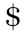Coding Mathematics : Fluid Flow Simulation

User Rating 4.9 (Total 489 votes)

Where am I going to use all the mathematics that I am studying? - is the most common question majority of students have. Some ask. Some don't. And then because of lack of purpose and direction, Mathematics becomes one fo their dreaded subjects. To demonstrate utility of all the engineering mathematics, software can be used very effectively. That too if we teach them - how to create software code for the mathematical concepts.

This booklet touches upon differential equations, especially for fluid flow. And then teaches step-by-step, how to create software code and visualize graphically all different kinds of fluid flows. It dives deep into mathematical model of fluid flow. We explore 3 case studies (a) Laminar flow, (b) Steady flow around cylinder, and (c) Vortex flow around cylinder. Every case starts with its respective theoretical model and mathematical discussion. Then how to translate those mathematical entities into software code. C++ has been chosen to be programming language for implementation. It is object oriented programming language, so design becomes elegant. Software architecture also becomes more polished as compared to procedural programming language.

1. Mathematical Model of Fluid Flow
2. Laminar Flow
3. Flow Around Cylinder
4. Vortex Flow
5. Software Model of Fluid Flow
6. Coordinate Systems and Mapping
7. Translate Equations into Mathematics
8. Design Pattern for Fluid Flow
9. Writing Functions in C++
10. Smooth Graphics Animation with Double Buffering
11. Developing GUI
12. Corollary : Line Source Fluid Flow
13. Corollary : Fluid Flow in Sink
14. 2000+ Lines of C++ Code
15. Runnable Demonstrations of 6 Fluid Flows Covered in Booklet

Pages : 32Fluid Flow Simulation is a Great Book. I purchased it a few days ago and loved it.

5.0

- Prof. Bhavna Joshi

Junior Research Fellow Computational Fluid Dynamics, Symbiosis University, Maharashtra, Pune33
 Quantity